Physics ELEMENTS OF CALCULUS
Click for Only Video 1

# Differentiation Basics

Click for Only Video 2

# Integration Basics

### Topics Covered• Intuitive Idea of Derivatives
• Limit
• Differential Calculus
• Integral Calculus

### Intuitive Idea of Derivatives

Physical experiments have confirmed that the body dropped from a tall cliff covers a distance of 4.9t^2 metres in t seconds, i.e., distance s in metres covered by the body as a function of time t in seconds is given by s = 4.9t^2.

The adjoining Table 13.1 gives the distance travelled in metres at various intervals of time in seconds of a body dropped from a tall cliff.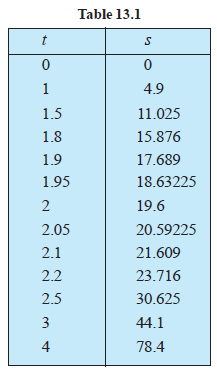The objective is to find the veloctiy of the body at time t = 2 seconds from this data. One way to approach this problem is to find the average velocity for various intervals of time ending at t = 2 seconds and hope that these throw some light on the velocity at t = 2 seconds.

Average velocity between t = t_1 and t = t_2 equals distance travelled between t = t_1 and t = t_2 seconds divided by (t_2 – t_1). Hence the average velocity in the first two seconds

= ( "Distance travelled between "t = t_2" and "t = t_1 ) /["Time interval " (t_2 – t_1)]

= [(19.6-0)m]/[ (2-0)s]= 9.8 m//s

Similarly, the average velocity between t = 1 and t = 2 is

= [(19.6-4.7)m]/[ (2-1)s]= 14.7 m//s

Likewise we compute the average velocitiy between t = t_1 and t = 2 for various t_1. The following Table 13.2 gives the average velocity (v), t = t_1 seconds and t = 2 seconds.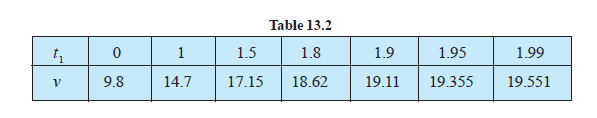From Table 13.2, we observe that the average velocity is gradually increasing.

Hoping that nothing really dramatic happens between 1.99 seconds and 2 seconds, we conclude that the average velocity at t = 2 seconds is just above 19.551m//s.

Compute the average velocities for various time intervals starting at t = 2 seconds. As before the average velocity v between t = 2 seconds and t = t_2 seconds is

= ( "Distance travelled between 2 seconds and seconds " ) /["Time interval " (t_2 –2 )]

= ( "Distance travelled in seconds - Distance travelled in 2 seconds " ) / (t_2 –2 )

The following Table 13.3 gives the average velocity v in metres per second between t = 2 seconds and t_2 seconds.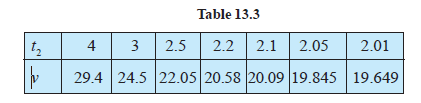In the first set of computations, what we have done is to find average velocities in increasing time intervals ending at t = 2 and then hope that nothing dramatic happens just before t = 2.

In the second set of computations, we have found the average velocities decreasing in time intervals ending at t = 2 and then hope that nothing dramatic happens just after t = 2.

Purely on the physical grounds, both these sequences of average velocities must approach a common limit.

We can safely conclude that the velocity of the body at t = 2 is between 19.551m//s and 19.649 m//s.

Technically, we say that the instantaneous velocity at t = 2 is between 19.551 m//s and 19.649 m//s.

color{green} "As is well-known, velocity is the rate of change of distance."

From the given data of distance covered at various time instants we have estimated the rate of change of the distance at a given instant of time. We say that the derivative of the distance function s = 4.9t^2 at t = 2 is between 19.551 and 19.649.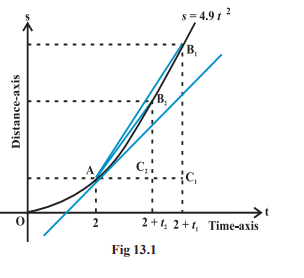In the limit as the sequence of time intervals h1, h2 approaches
zero, the sequence of average velocities approaches the same limit as does the sequence of ratios

(C_1B_1)/(AC_1), (C_2B_2)/(AC_2),(C_3B_3)/(AC_3)

### LIMITConsider the function f(x) = x^2.. Observe that as x takes values very close to 0, the value of f(x) also moves towards 0

lim_(x->0)f(x) = 0

(to be read as limit of f (x) as x tends to zero equals zero).The limit of f (x) as x tends to zero is to be thought of as the value f (x) should assume at x = 0.

In general as x → a, f (x) → l, then l is called limit of the function f (x) which is symbolically written as lim_(x->a)f(x) = l.

### Differential Calculus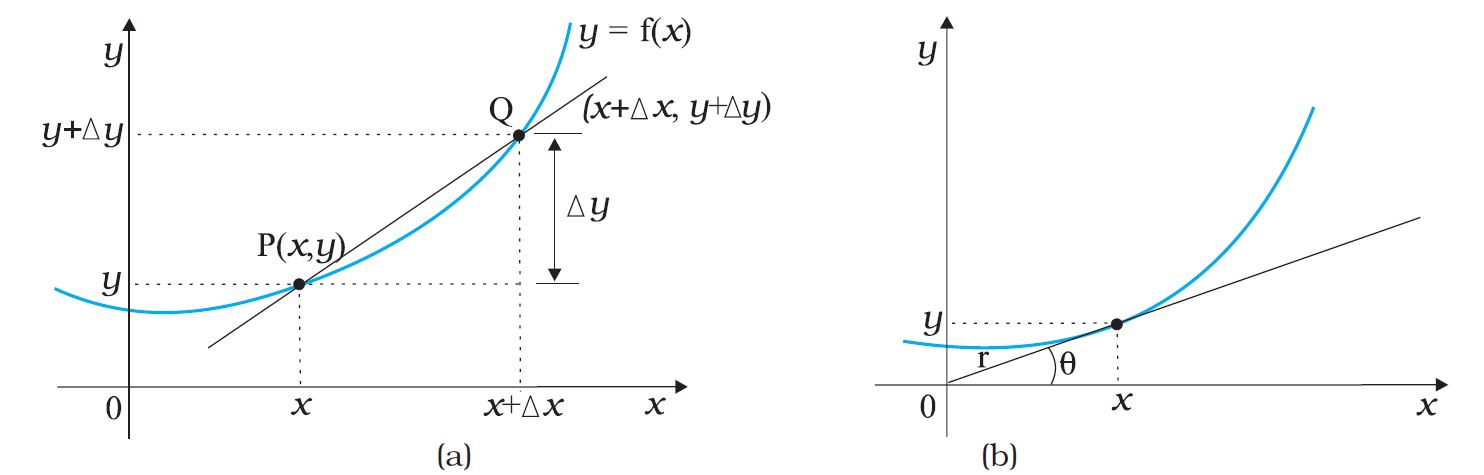Suppose y=f(x)

This relationship can be visualised by drawing a graph of function y=f(x) as shown in Fig. (a).

Consider the point P(x,y) and point Q(x+Deltax, y+Deltay) on the curve y=f(x).
Slope of line joining PQ : tan theta=(Deltay)/(Deltax)=((y+Deltay)-y)/(Deltax)

Suppose now that the point Q moves along the curve towards P. In this process, Deltay->0, Deltax->0; though their ratio (Deltay)/(Deltax) will not necessarily vanish. This line becomes a tangent to the curve at point P as shown in Fig. (b).

This means that tan theta approaches the slope of the tangent at P, denoted by m:
m=lim_(Deltax->0) (Deltay)/(Deltax) = lim_(Deltax->0) ((y+Deltay)-y)/(Deltax)

• The limit of the ratio Δy/Δx as Δx approaches zero is called the derivative of y with respect to x and is written as dy/dx.

• It represents the slope of the tangent line to the curve y = f (x) at the point (x, y).

Since y = f (x) and y + Δy = f (x + Δx), we can write :

(dy)/(dx)=(df(x))/(dx)=lim_(Deltax->0) (Deltay)/(Deltax)=lim_(Deltax->0)[(f(x+Deltax)-f(x))/(Deltax)]

### Integral Calculus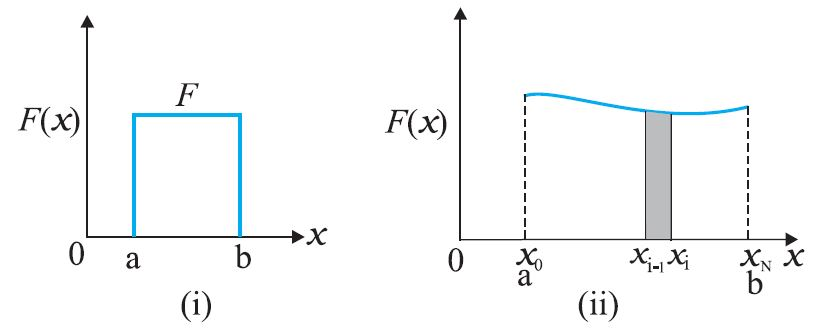Suppose a variable force f (x) acts on a particle in its motion along x - axis from x = a to x = b. The problem is to determine the work done (W) by the force on the particle during the motion.

• If the force were constant, work would be simply the area F (b-a) as shown in Fig. (i).

• If force is varying, To calculate the area under this curve [Fig. (ii)], divide the interval on x-axis from a to b into a large number (N) of small intervals: x_0(=a) to x_1, x_1 to x_2, x_2 to x_3, ............x_(N-1) to x_N(=b).

The area under the curve is thus divided into N strips. Each strip is approximately a rectangle, since the variation of F(x) over a strip is negligible.

The area of i^(th) strip is approximately DeltaA_i=F(x_i) (x_i -x_(i-1))=F(x_i) Deltax
The total area under the curve A=sum_(i=1)^N (DeltaA_i) = sum_(i=1)^N F(x_i) Deltax

The limit of this sum as N->oo is known as the integral of F(x) over x from a to b. It is given a special symbol as shown below:
A=int_a^b F(x) dx

• A most significant mathematical fact is that integration is, in a sense, an inverse of differentiation.

Suppose we have a function g (x) whose derivative is f (x), i.e. f(x)=(dg(x))/(dx)
The function g (x) is known as the indefinite integral of f (x) and is denoted as: g(x)=intf(x)dx

• An integral with lower and upper limits is known as a definite integral. It is a number.
• Indefinite integral has no limits; it is a function.

• A fundamental theorem of mathematics states that int_a^b f(x)dx=g(x)|_a^b =g(b)-g(a)

### Differential Calculus ( Formula)

d/(dx) (sinx ) = cos x \ \ \ \ : \ \ \ \ d/(dx) (cos x ) = - sin x

d/(dx) (tan x) = sec ^2x \ \ \ \ : \ \ \ \ d/(dx) (cot x ) = - cosec^2x

d/(dx) (sec x ) = tan x sec x \ \ \ \ : \ \ \ \ d/(dx) (cosec^2x ) = - cot x cosec x

d/ (du) ( e^u) = e^u

color(blue)(★ul"Multiplication by constant")

(d (a u ) )/(dx) = a (du ) /(dx) \ \ \ \ : \ \ \ \ (du )/(dt) = (du)/(dx) * (dx)/(dt)

color(blue)(★ul"Power Rule ")

d/(dx) (u)^n = n u ^(n -1) (du)/ (dx) \ \ \ \ : d/ (du ) ( ln u ) = 1/u

d/(dx) ( x^n) = nx^(n-1)

color{green}"Example :" f(x) = 8 x^5 + 4x^4 + 6^3 + x +7

d/(dx)f(x) =f'(x) = 8 (5)x^(5-1) + 4 (4) x ^(4-1) + 6(3)x^(3-1) + x^(1-1)

f'(x) = 40 x^4 + 16x^3 + 18x^2 +1

color(blue)(★ul"Product Rule")

(d(uv))/(dx) = u (dv)/(dx) + v (du)/(dx)

color{green}"Example :" f(x) = x^3 cosx

d/(dx)f(x) =f'(x) = x^3 d/(dx) (cos x) +(cos x) d/(dx) (x^3 )

f'(x) = x^3 ( -sinx ) + 3 x^2 cos x

color(blue)(★ul"Quotient Rule ")

(d(u//v))/(dx) = [v (du)/(dx) - u (dv)/(dx)]/v^2

color{green}"Example 1 :" f(x) = x /(x +1)

d/(dx)f(x) = f'(x) = (( x+1) (d(x))/(dx) - (x) (d)/(dx)(x+1) )/(x+1 )^2 = 1/ (x +1)^2

color{green}"Example 1 :" f(x) = x^2/(sinx)

d/(dx)f(x) = f'(x) = ( sin x(d)/(dx) (x^2) - (x^2)(d)/(dx)(sinx))/(sinx)^2 = (2x sin x - x^2cos x)/(sin^2x)

(du)/(dv) = (du//dx)/(dv//dx)

### Integral Calculus (Formula )

color{blue}{ int x^n dx = (x^(n +1)) / (n +1) }\ \ \ \ \ \ (n != -1 )

color{green}"Example :" f(x) = x^4 + 3x^2 + x +1

int f(x) dx = 8 int x^4 dx + 3 int x^2 dx + int x dx + int 1 dx

int f(x) dx= (8x^5)/5 + (3x^3)/3 + x^2/2 +x

int f(x) dx= 8/5x^5 +x^3 + 1/2x^2 +x

color{blue}{ int (1/x) dx = ln x} \ \ \ \ \ \ ( x > 0 )

color{blue}{ int sin x dx = - cos x \ \ \ \ \ \ int cos x dx = sin x}

color{blue}{ int e^x dx = e^x}